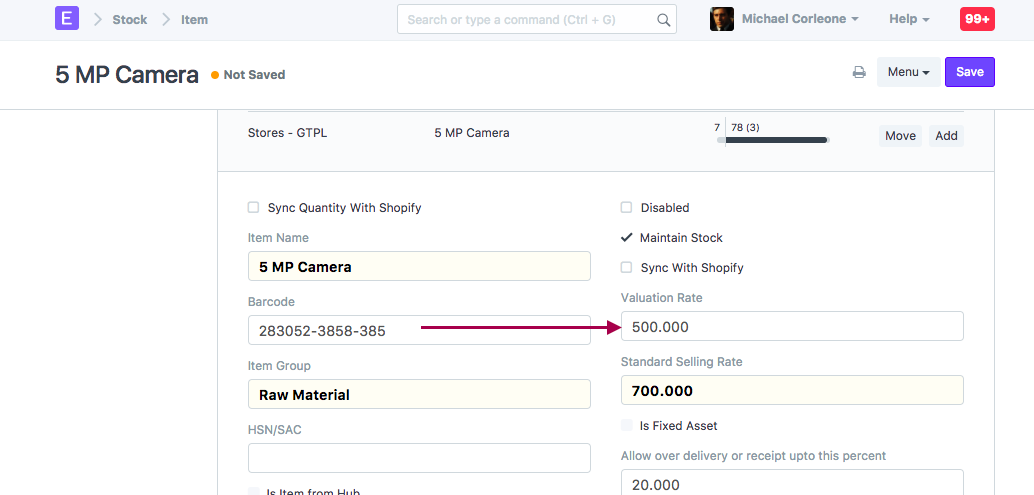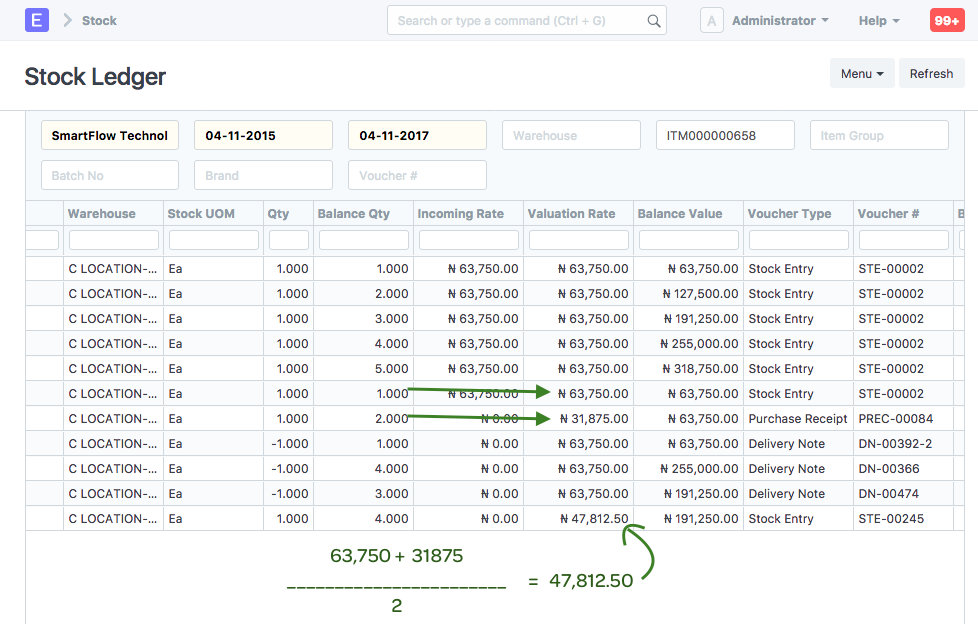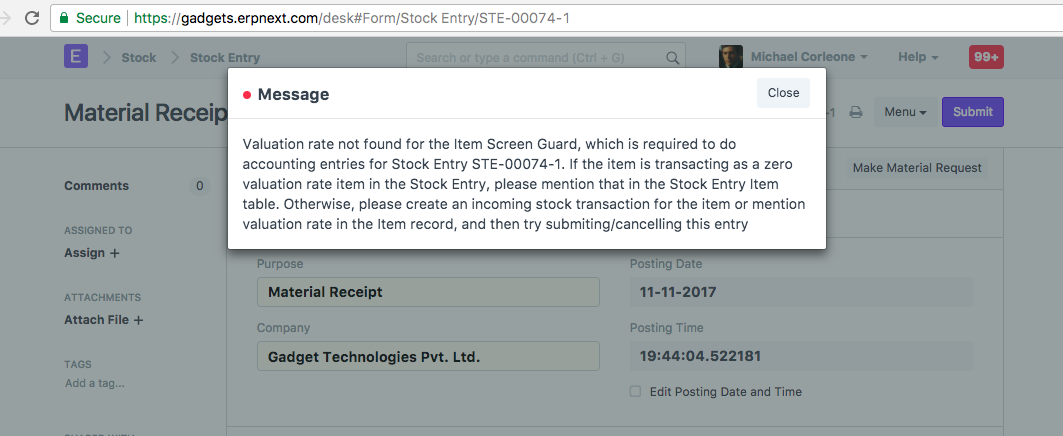###### By Umair Sayed on November 11, 2017
Intermediate

When item's stock is inwarded via stock transactions (like Purchase Receipt, Stock Entry or Stock Reconciliation), valuation is calculated for an item. There are the various sources of rates based on which valuation rate is calculated. Following are the options which explain various cases where rates are picked from different sources for calculating the item's valuation rate.

1. Based on the Basic Rate / Incoming Rate / Valuation Rate entered in an entry, valuation rate is calculated for an item. In the transactions, you can enter item's rate, apply taxes and other charges. Based on these values, Valuation Rate is calculated for an item.

2. If the user doesn't enter any valuation rate, then it checks for Valuation Rate field in the Item master, in the first section.3. If both the above fail to provide valuation rate to an item, then it calculates the average of old valuation rate available for this item and applies for the current stock entry. This is what happened in the Stock Entry in question.4. If there is no reference at all, also allowing stock entry at zero valuation is not enabled, then system correctly throws this validation message.